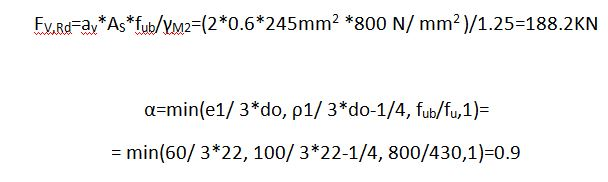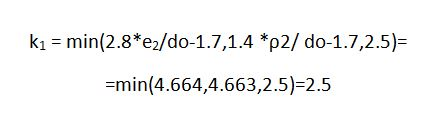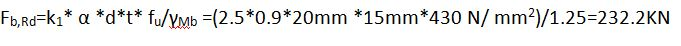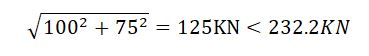Tuesday, 30 October 2018 13:36cat

## Calculation Example – Shear bolt connection EC3. Featured

Written by  TheStructuralEngineer.info

Check the shear connection for the IPE450-S275 for the design shear VEd=300KN. Bolts M20-8.8, plate thickness t=15mm.

e 1=60mm > 1.2*do=1.2*22=26.4mm

e 2=50mm > 1.2*do=1.2*22=26.4mm

e 1, e 2< 4*t+40=100mm

ρ1>2.2*do=2.2*22=48.4mm

ρ2>2.4*do=2.4*22=52.8mm

ρ1 and ρ2< min(14t,200)=210mmEvery bold gets vertical force 300/3=100KN and the torsional moment is 300KN*50mm=1500KN*cm and it is assigned at the farthest bolts as horizontal force 1500KN*cm/20cm=75KN.

At the farthest bolts the maximum force is